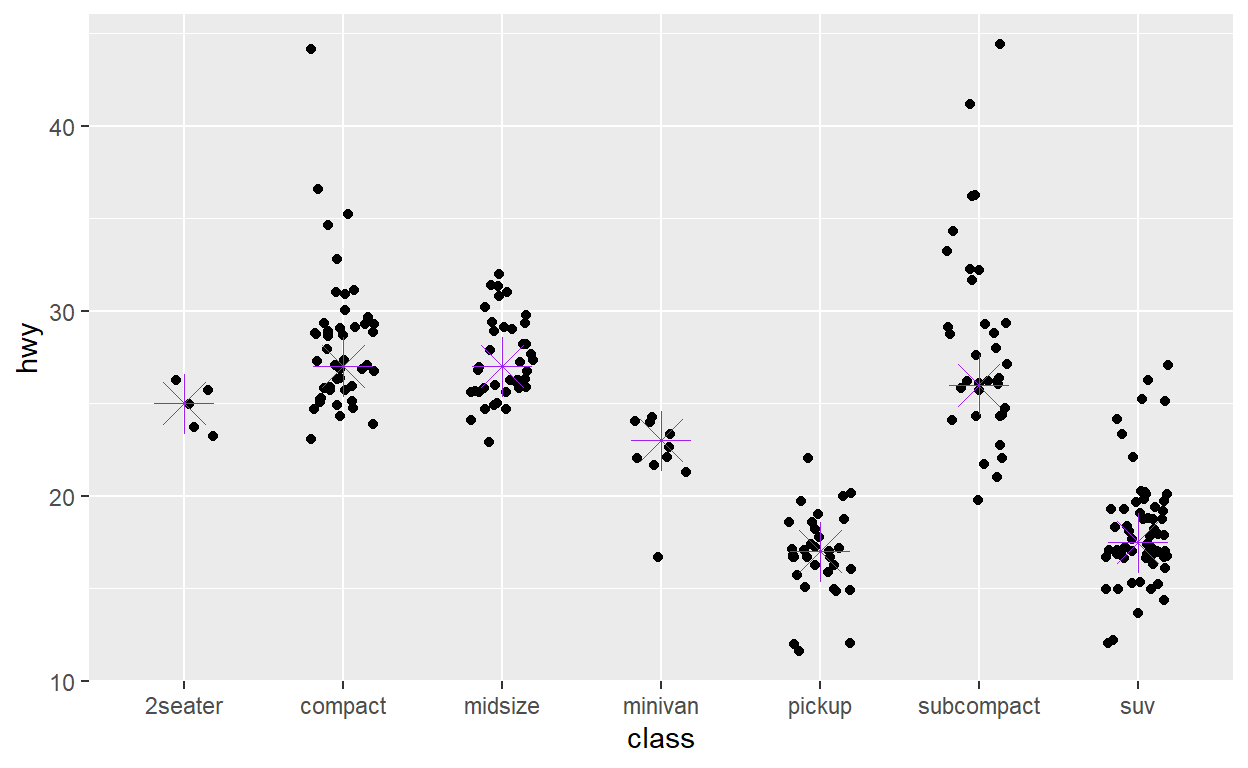# Exploratory analysis

A short description of the post.

``````library(tidyverse)
``````

# Introduction

``````data("faithful")
# Basic Scatterplot

ggplot(data = faithful,
mapping = aes(x = eruptions, y = waiting)) +
geom_point()
``````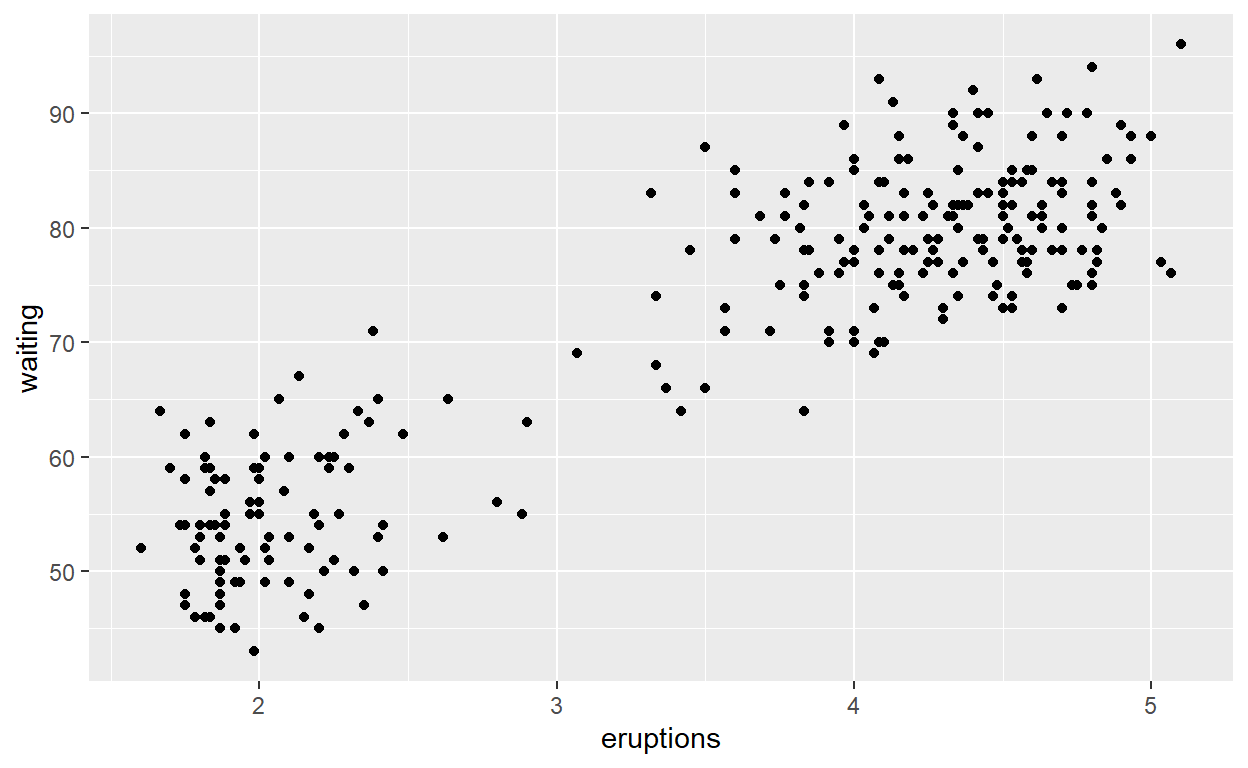``````# Data and ampping can be given both as global (in ggplot()) or per layer

ggplot() +
geom_point(mapping = aes(x = eruptions, y = waiting),
data = faithful)
``````# 25 - 35

``````ggplot(faithful) +
geom_point(aes(x = eruptions,
y = waiting,
colour = eruptions < 3))
``````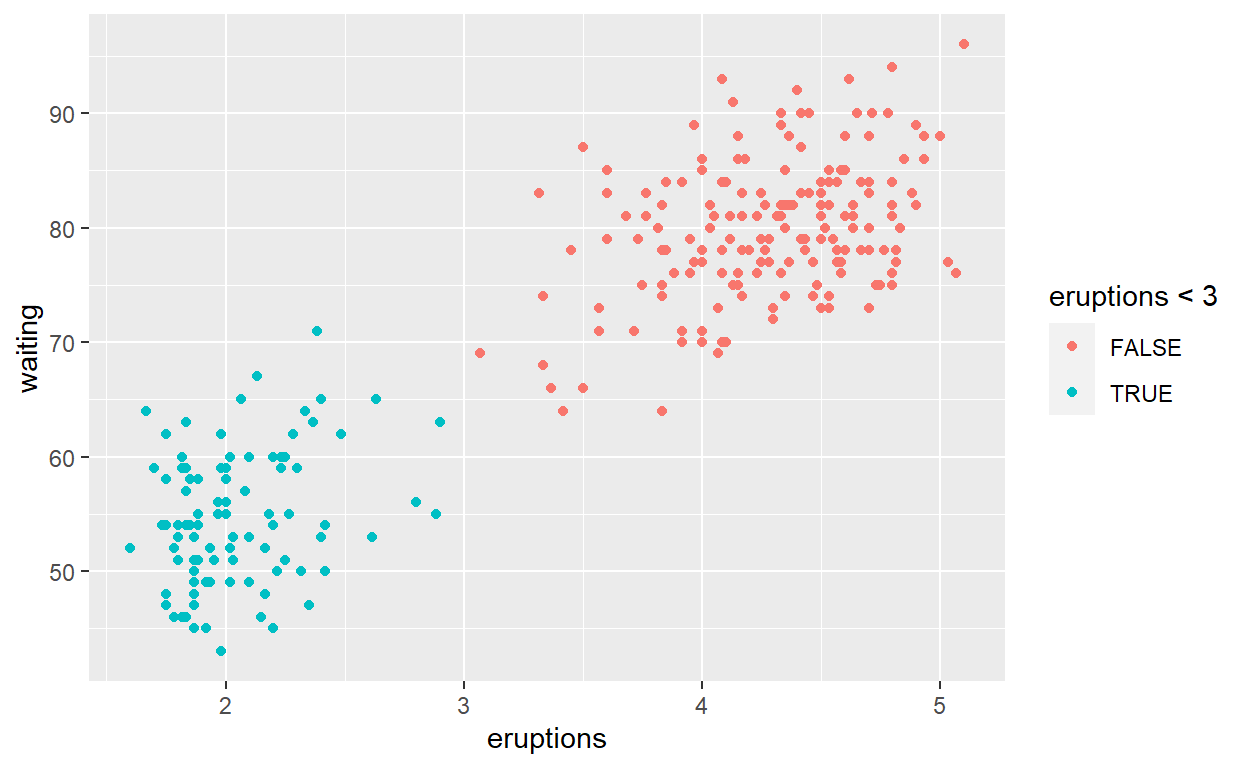simplify

``````ggplot(faithful) +
geom_point(aes( x = eruptions, y = waiting),
color = 'steelblue')
``````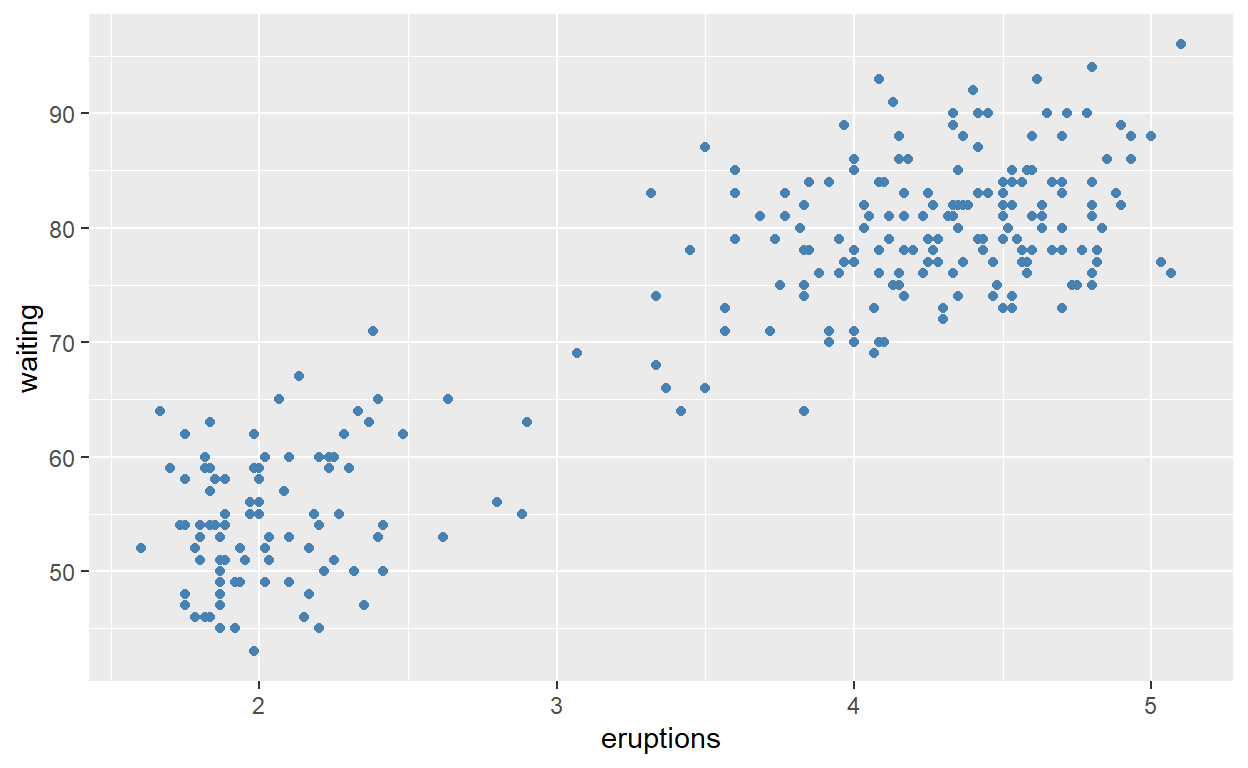Single mapping

``````ggplot(faithful) +
geom_histogram(aes(x = eruptions))
``````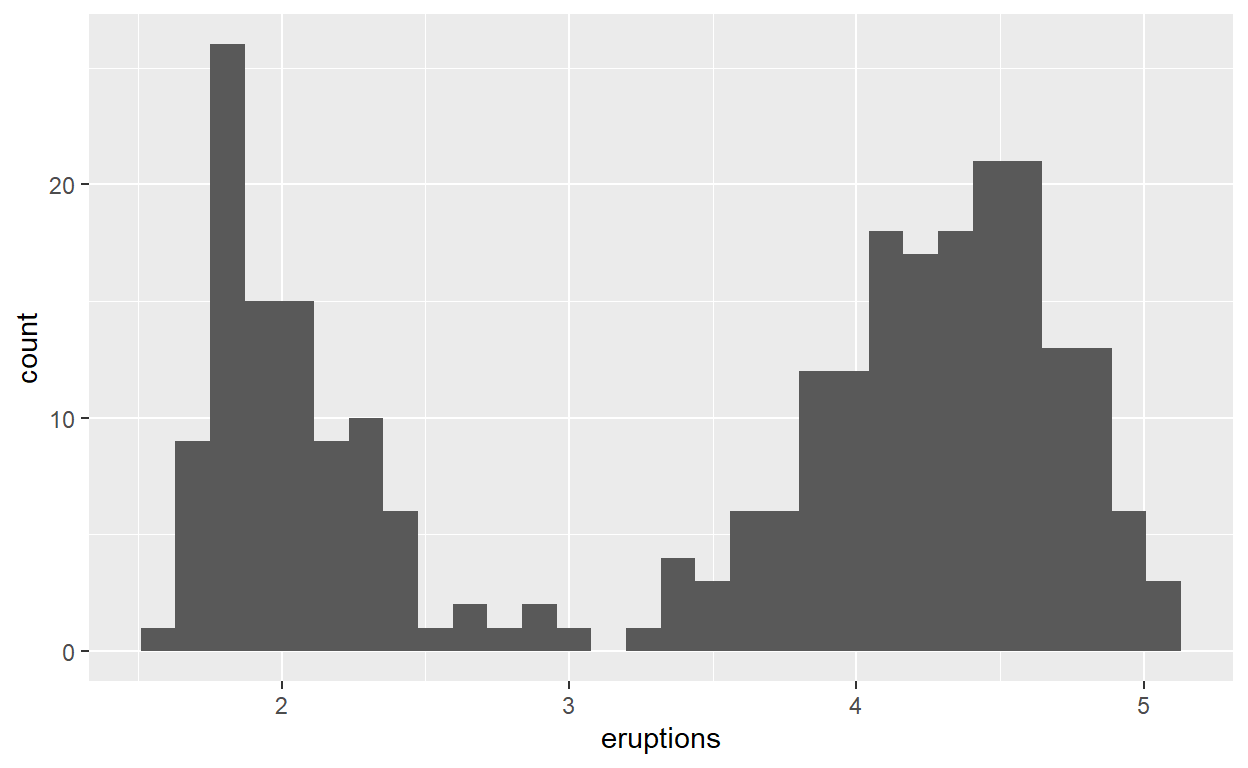# 36-38

Layers

``````ggplot(faithful,
aes(x = eruptions, y = waiting)) +
geom_density_2d() +
geom_point()
``````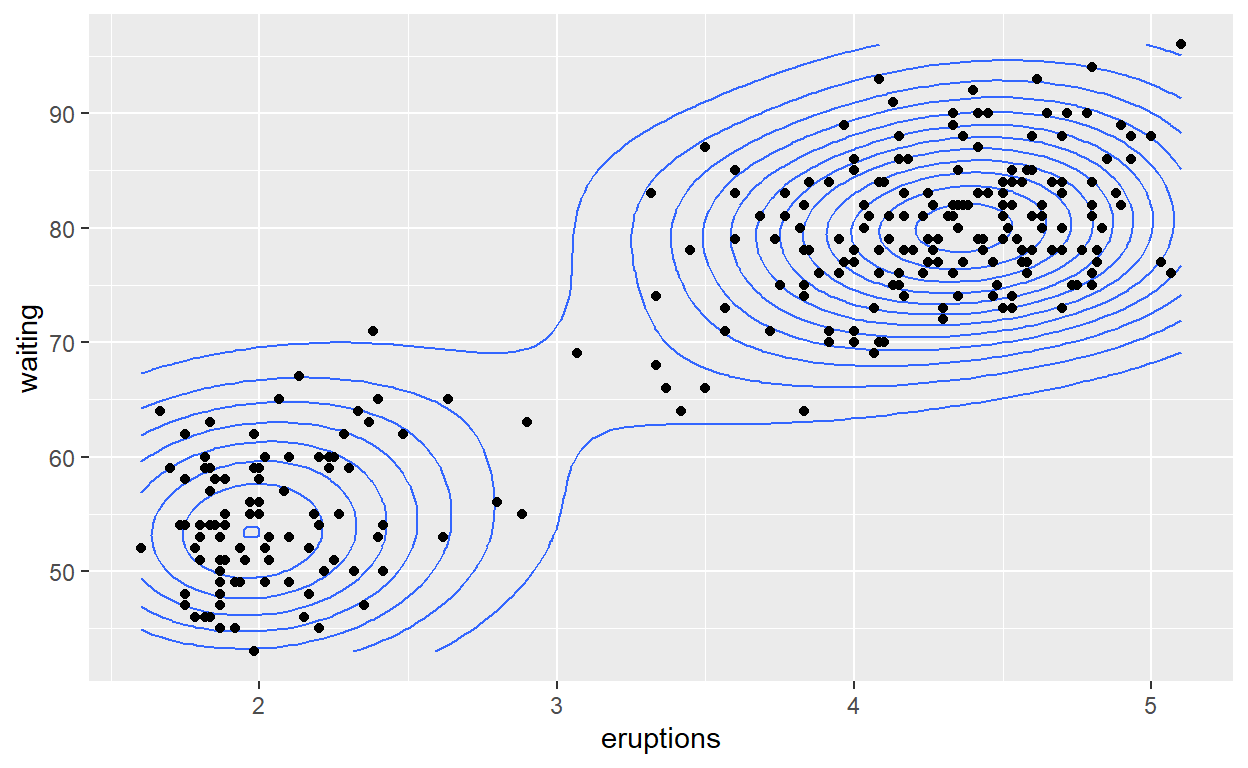Transparent

``````ggplot(faithful) +
geom_point(aes(x = eruptions, y = waiting), shape = 'square', alpha = 0.3)
``````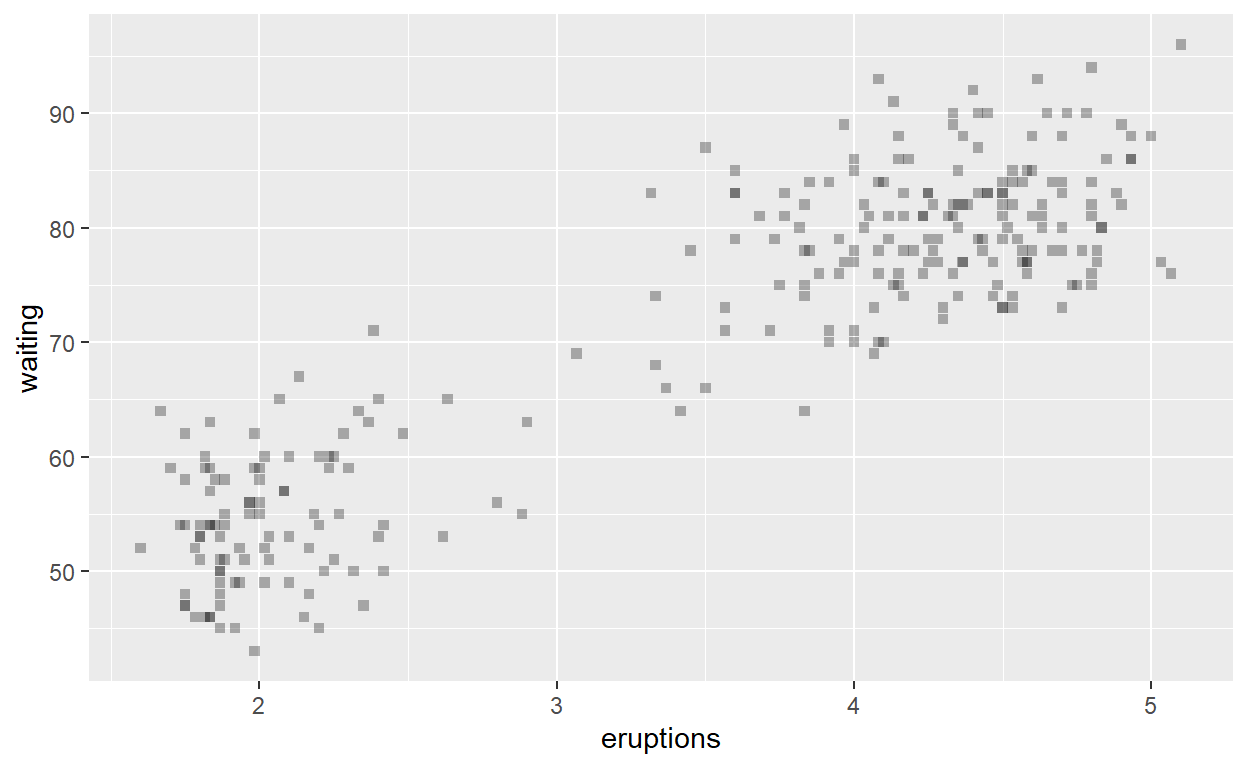``````ggplot(faithful) +
geom_histogram(aes( x = eruptions, fill = eruptions < 3.1))
``````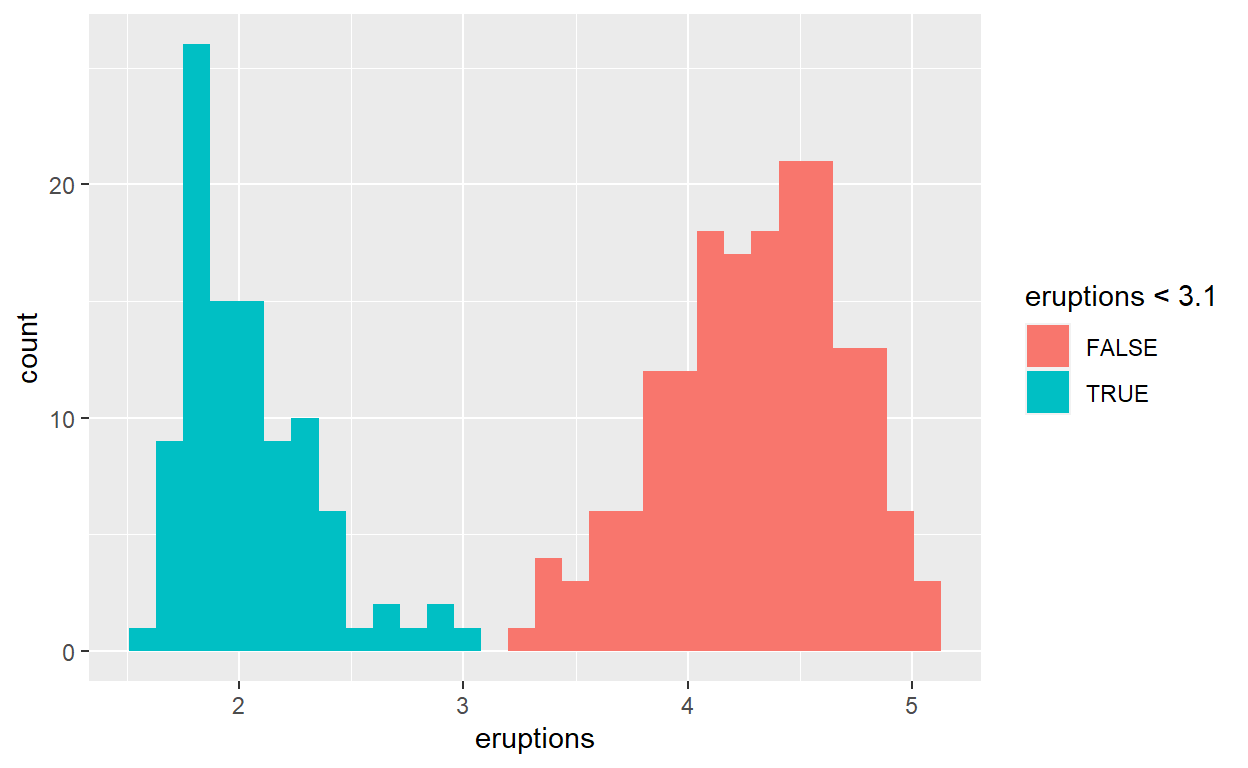``````ggplot(faithful) +
geom_histogram(aes(x = eruptions, fill = waiting < 60), position = 'dodge', alpha = 0.7)
``````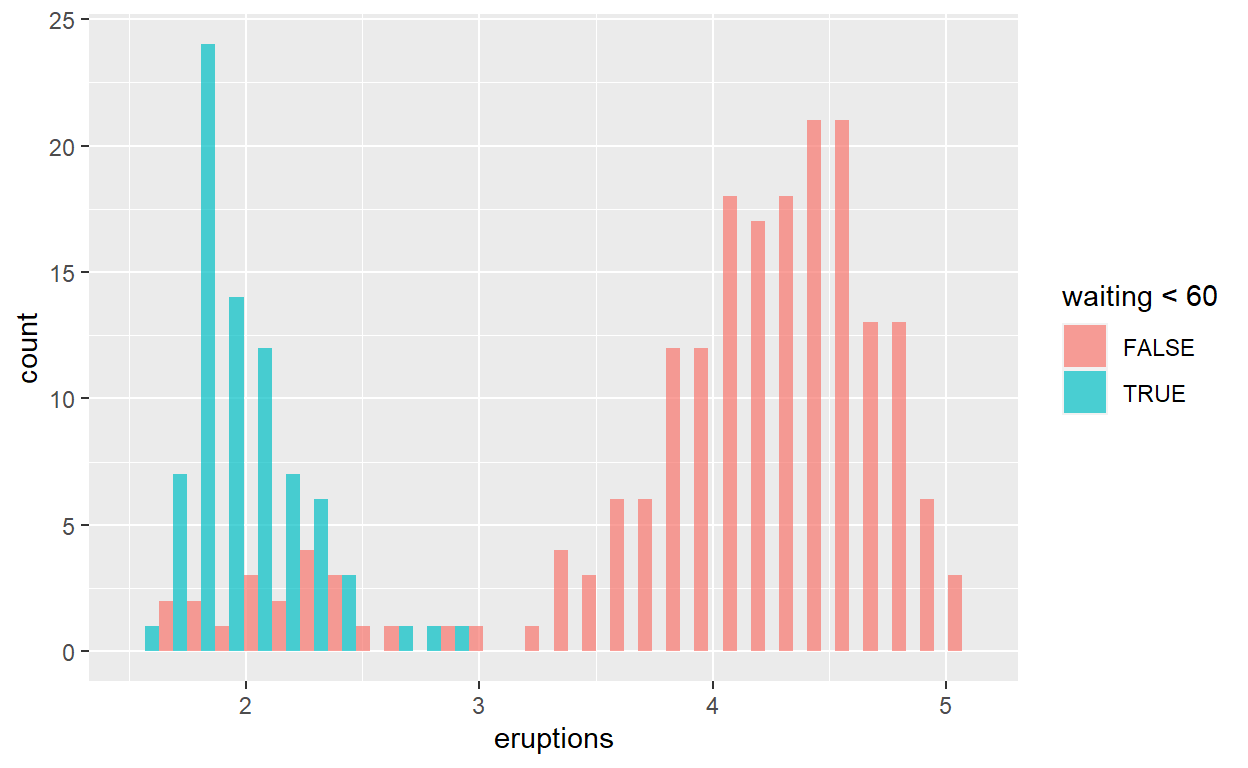Add a line that separates the two point distributions. See `?geom_abline` for how to draw straight lines from a slope and intercept.

``````ggplot(faithful) +
geom_point(aes(x = eruptions, y = waiting)) +
geom_abline(slope = -40, intercept = 200)
``````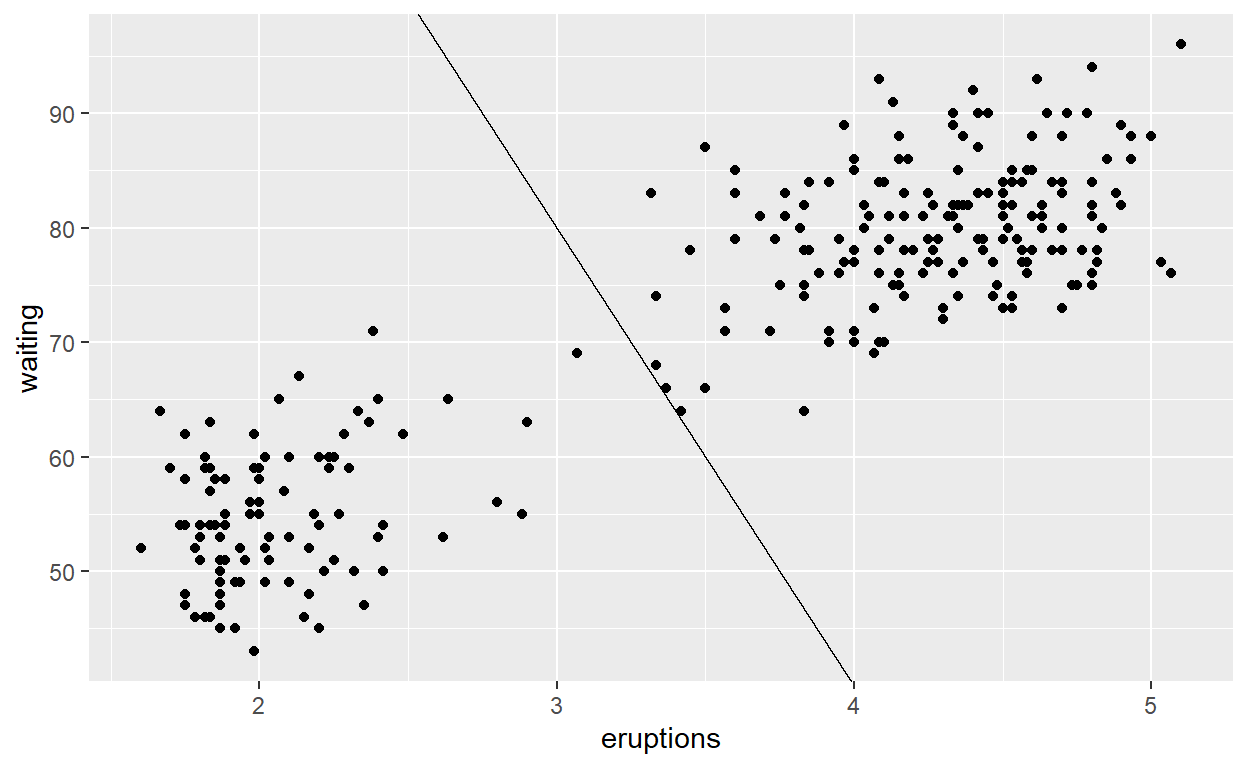# 39-45 slides

``````ggplot(mpg) +
geom_bar(aes(x = class))
``````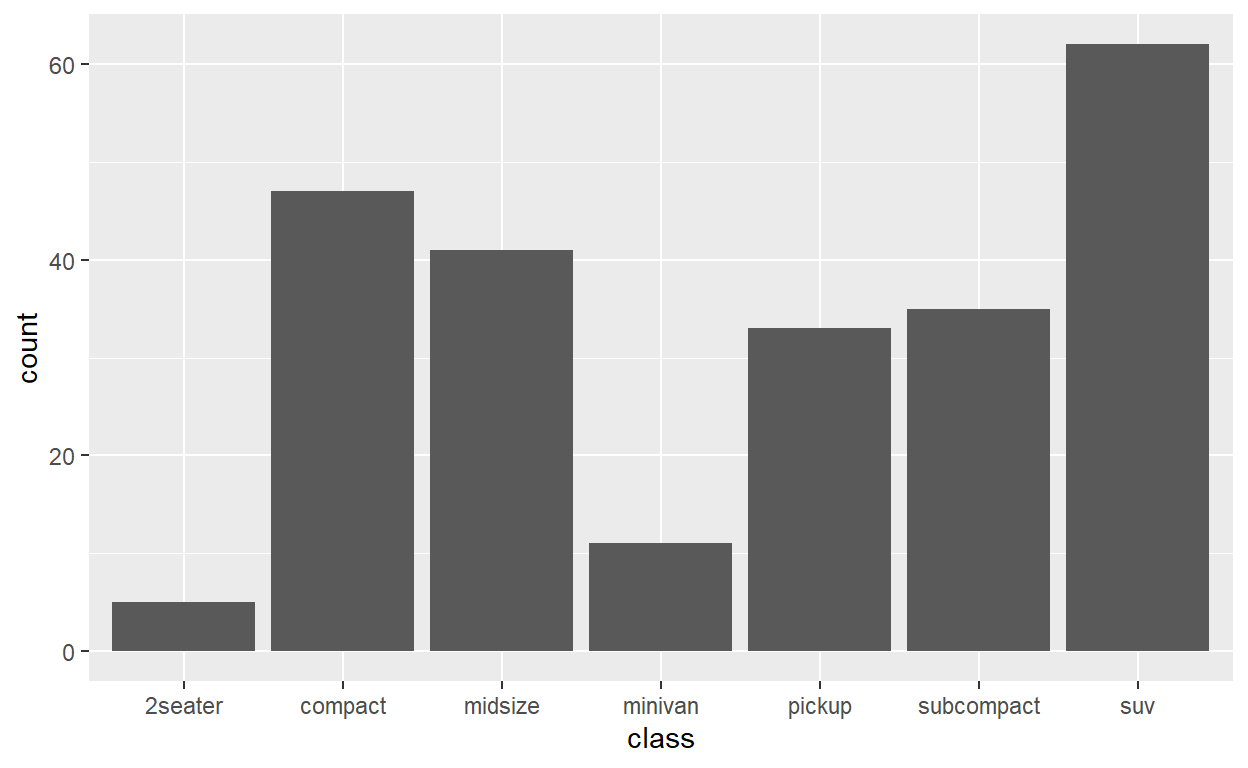``````mpg_counted <- mpg %>%
count(class, name = 'count')
``````
``````ggplot(mpg_counted) +
geom_bar(aes(x = class, y = count),
stat = 'identity')
``````Use `stat_summary()` to add a red dot at the mean `hwy` for each group

``````ggplot(mpg) +
geom_jitter(aes(x = class, y = hwy), width = 0.2) +
stat_summary(aes(x = class, y = hwy), fun = mean, geom = 'point', color = 'red', size = 2)
``````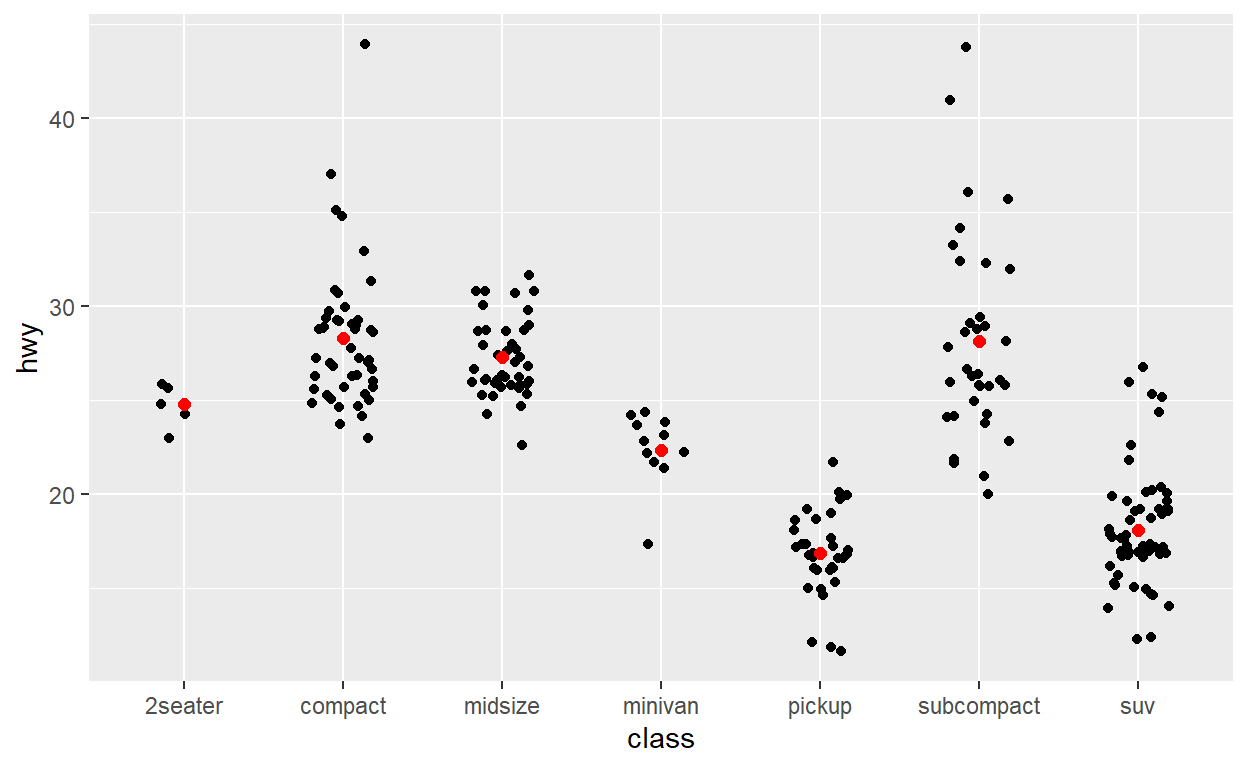``````ggplot(mpg) +
geom_point(
aes( x = displ, y = hwy, color = class)
)
``````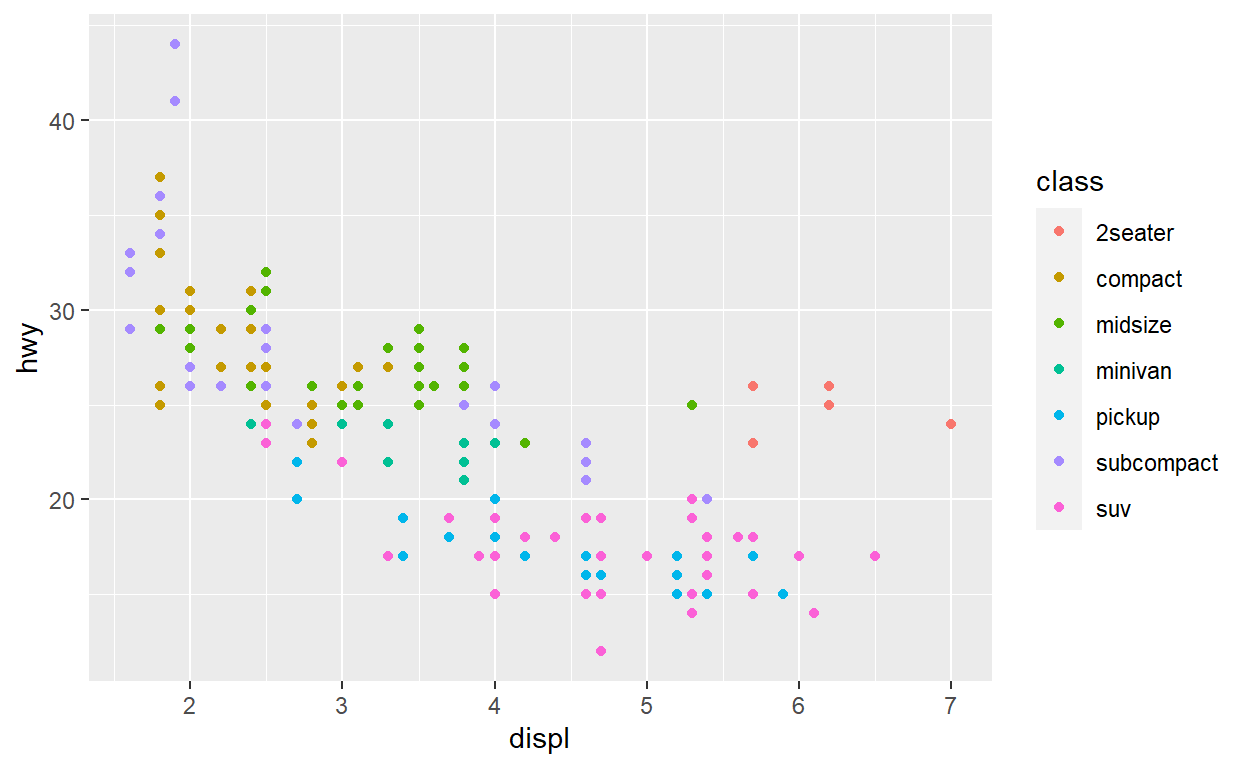``````ggplot(mpg) +
geom_point(
aes(x = displ, y = hwy, color = class)
) +
scale_color_brewer(name = "CLASS", type = 'qual')
``````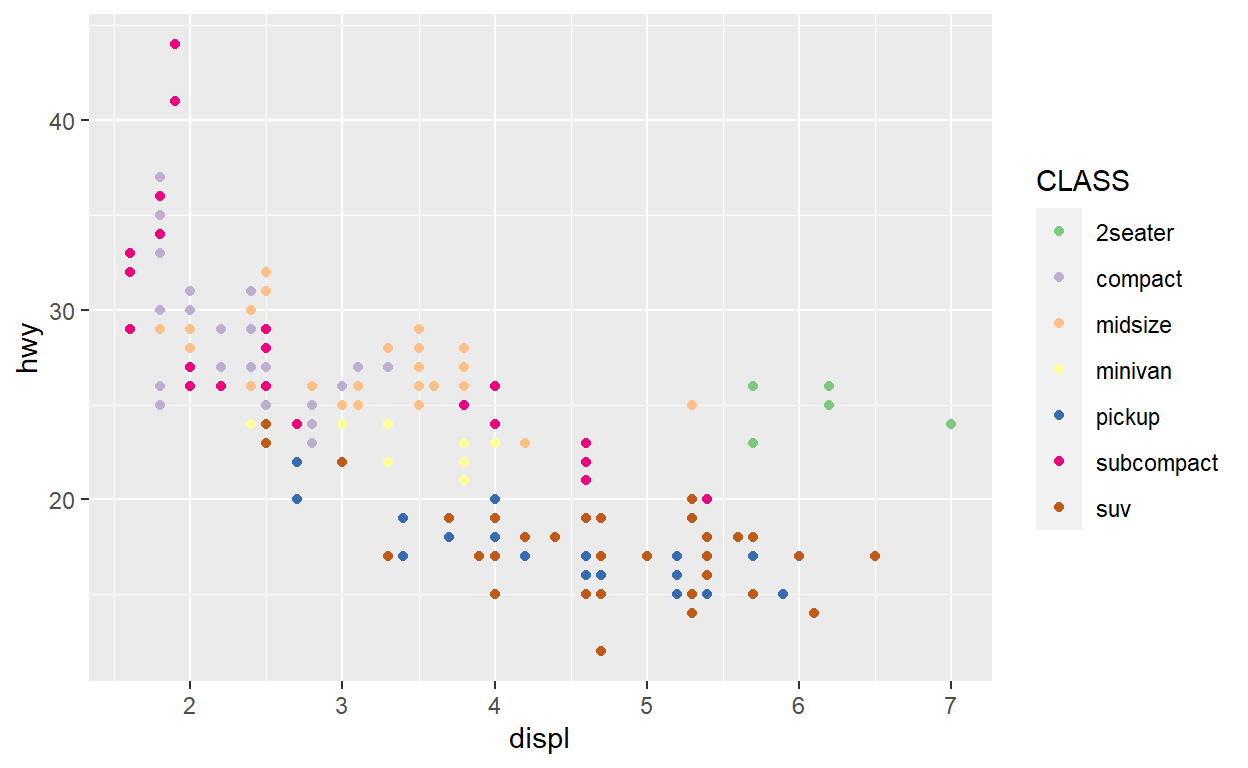# 49

``````ggplot(mpg) +
geom_point(aes(x = displ, y = hwy)) +
scale_x_continuous(breaks = c(3,5,6)) +
scale_y_continuous(trans = 'log10')
``````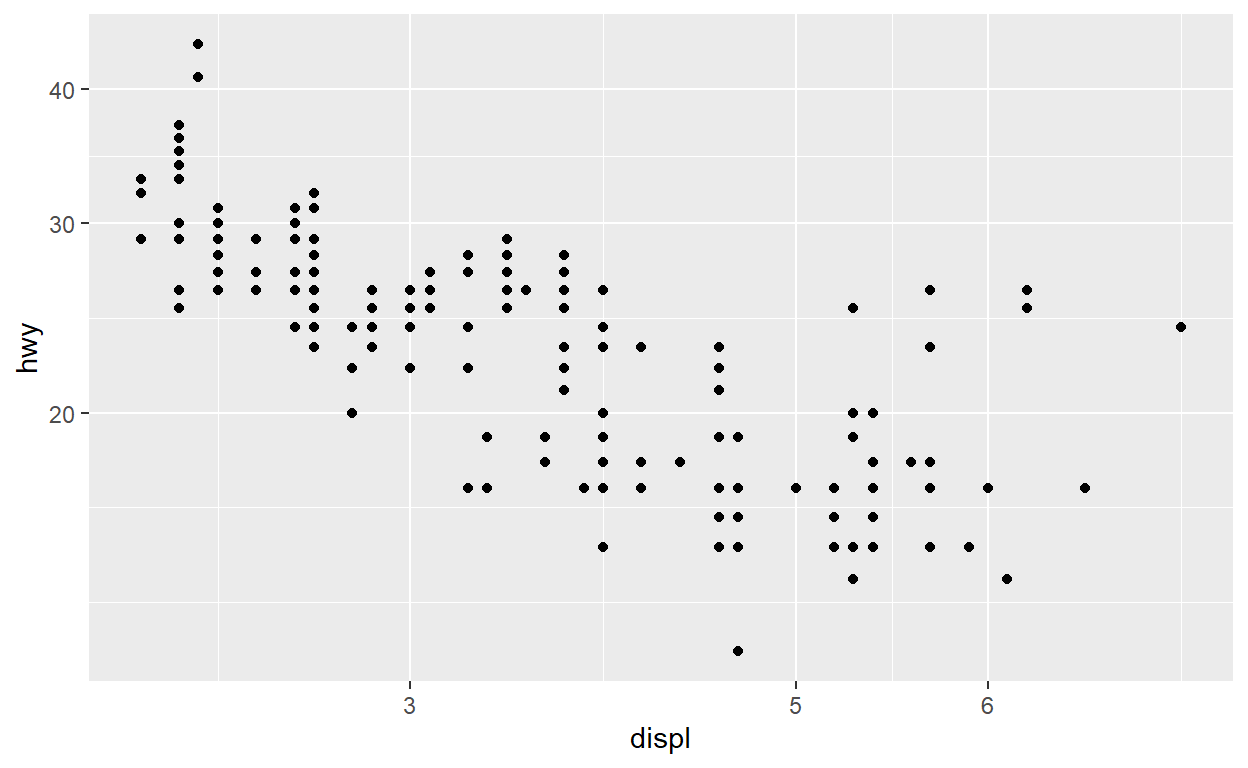#### Exercises

Use `RColorBrewer::display.brewer.all()` to see all the different palettes from Color Brewer and pick your favourite. Modify the code below to use it

``````ggplot(mpg) +
geom_point(aes(x = displ, y = hwy, color = class)) +
scale_color_brewer(palette = 'Set1')
``````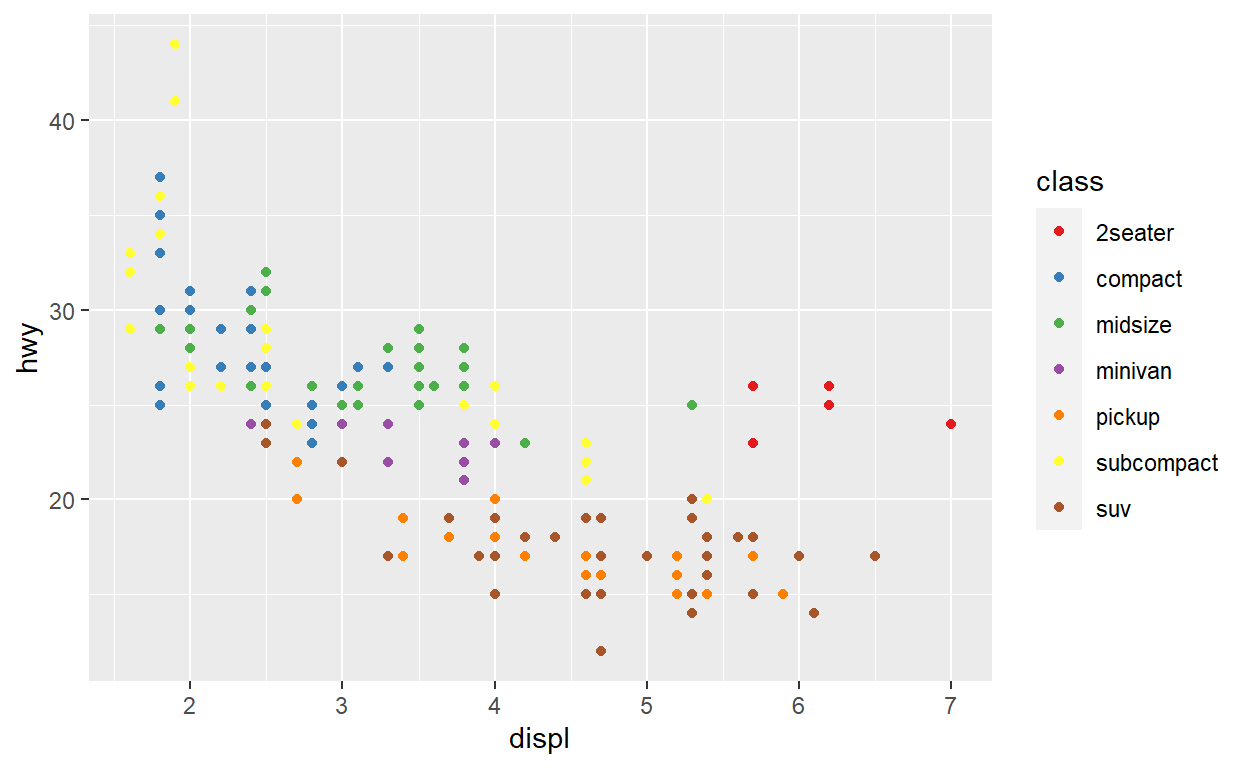Modify the code below to create a bubble chart (scatterplot with size mapped to a continuous variable) showing `cyl` with size. Make sure that only the present amount of cylinder (4,5,6, and 8) are present in the legend.

``````ggplot(mpg)+
geom_point(aes(x = displ, y = hwy, color = class, size = cyl)) +
scale_color_brewer(type = 'qual') +
scale_size_area(breaks = c(4,5,6,8))
``````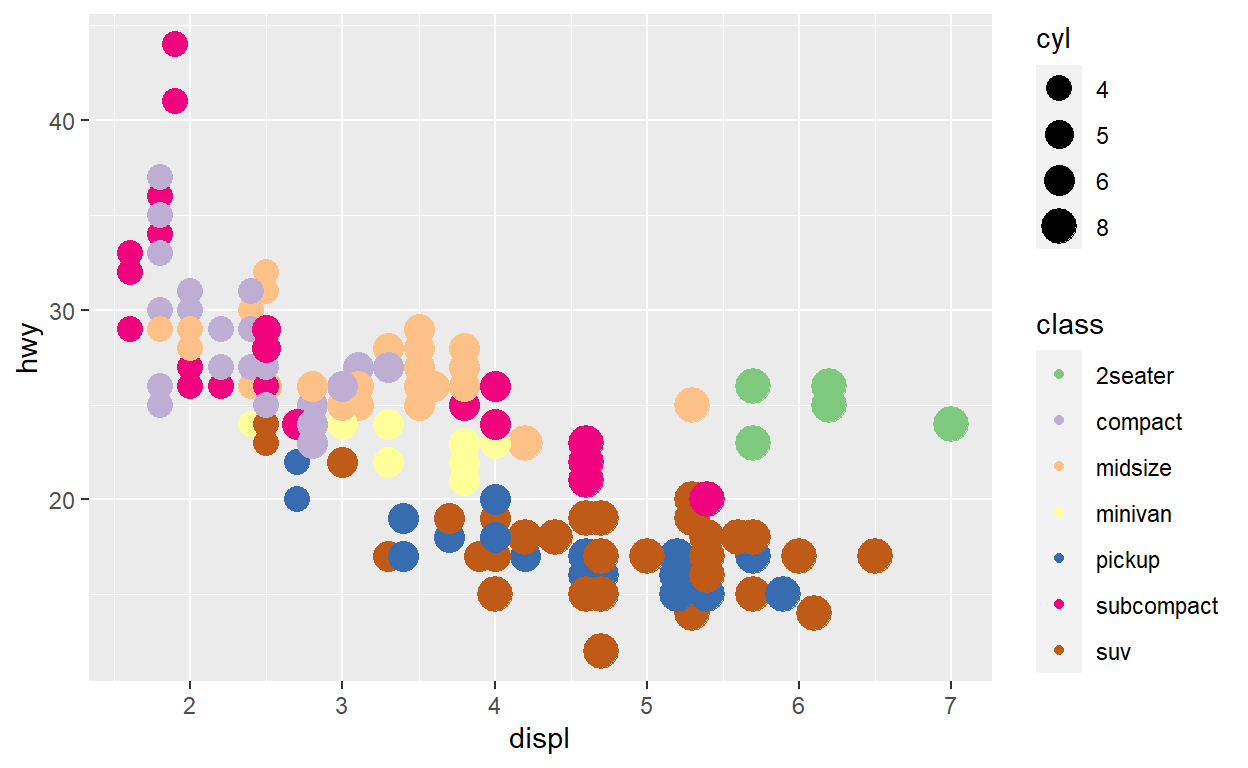Modify the code below so that colour is no longer mapped to the discrete `class` variable, but to the continuous `cty` variable. What happens to the guide?

``````ggplot(mpg) +
geom_point(aes(x = displ, y = hwy, color = cty, size = cty)) +
guides(color = 'legend')
``````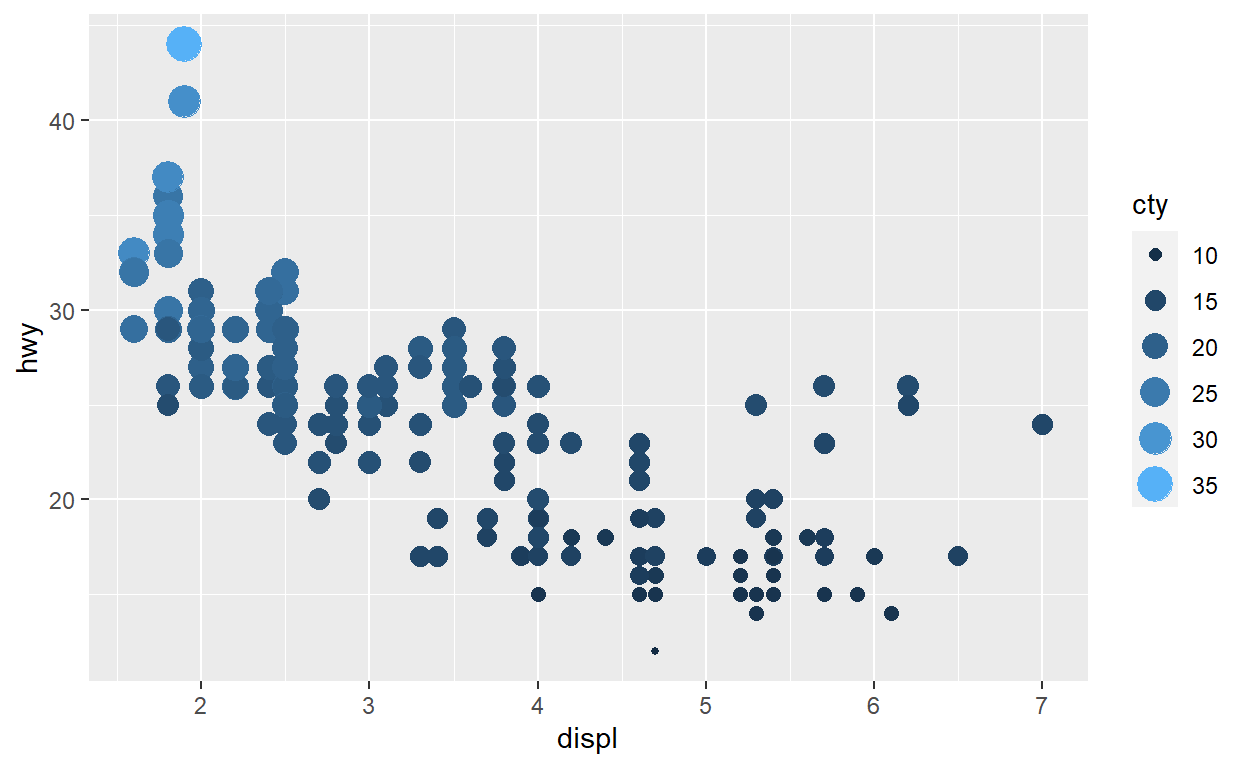**** Quiz Q1

``````ggplot(faithful) +
geom_point(aes(x = eruptions, y = waiting,
color = waiting > 77))
``````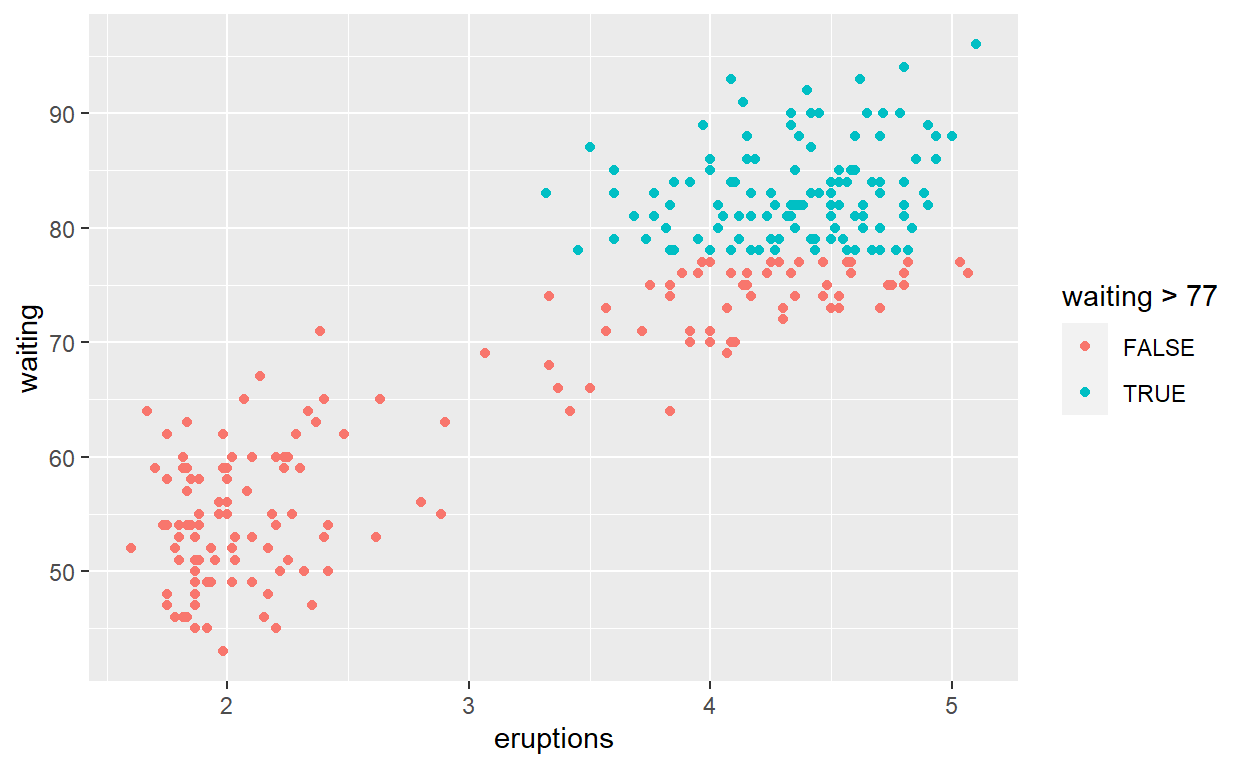# Quiz Q2

``````ggplot(faithful) +
geom_point(aes(x = eruptions, y = waiting), color = "blueviolet")
``````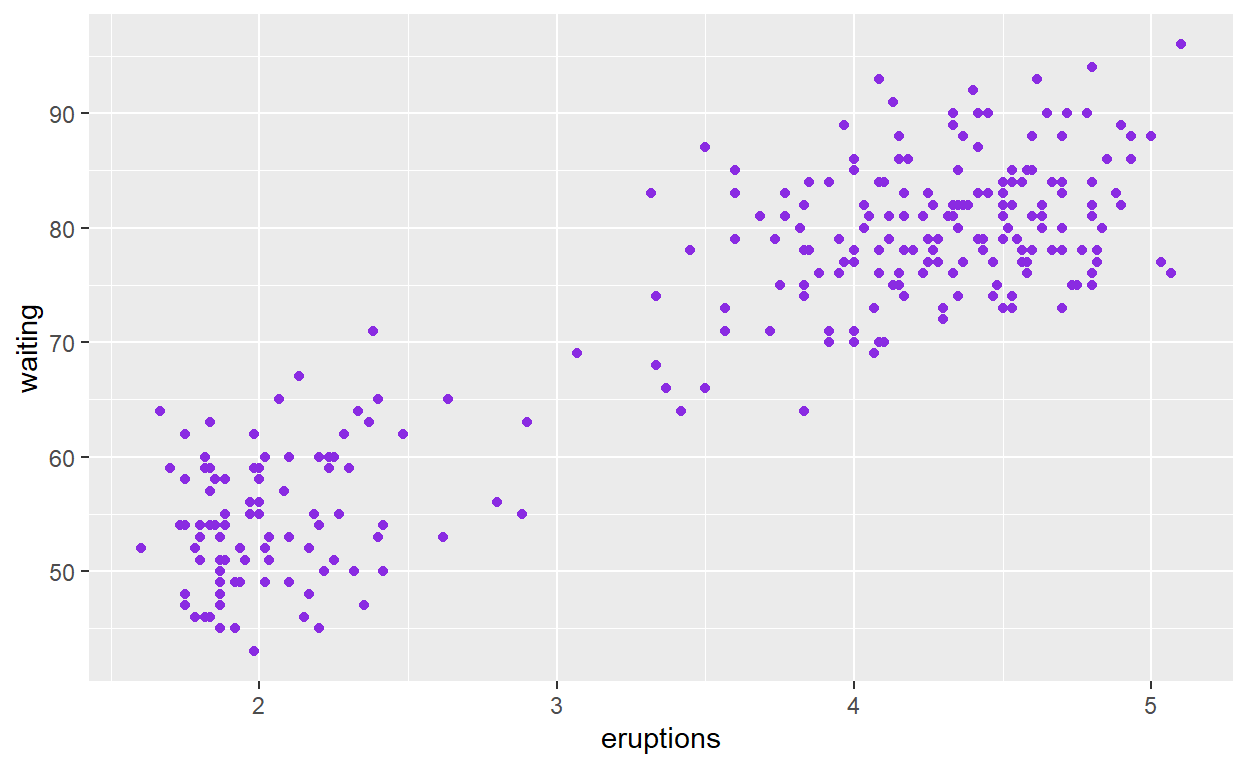# Quiz Q3

``````ggplot(faithful) +
geom_histogram(aes(x = waiting))
``````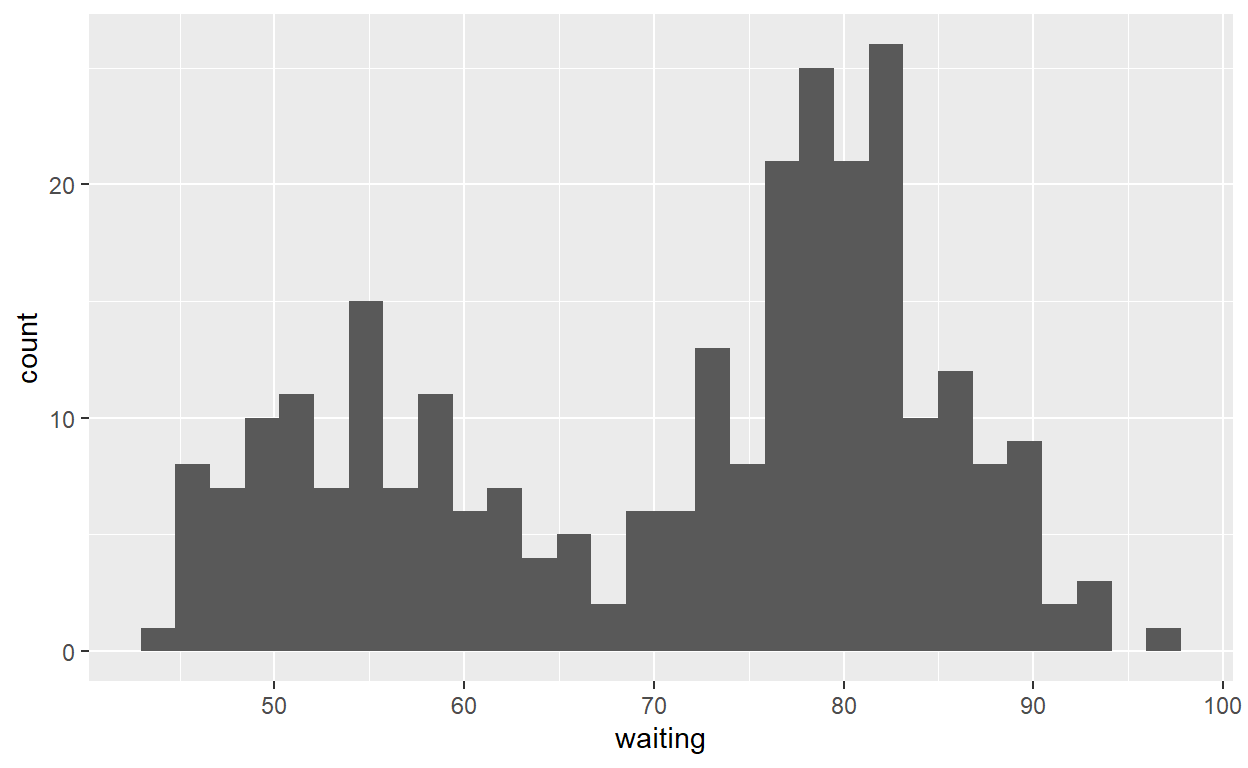# Quiz Q4

``````ggplot(faithful) +
geom_point(aes(x = eruptions, y = waiting),
shape ="cross", size = 4, alpha = 0.3)
``````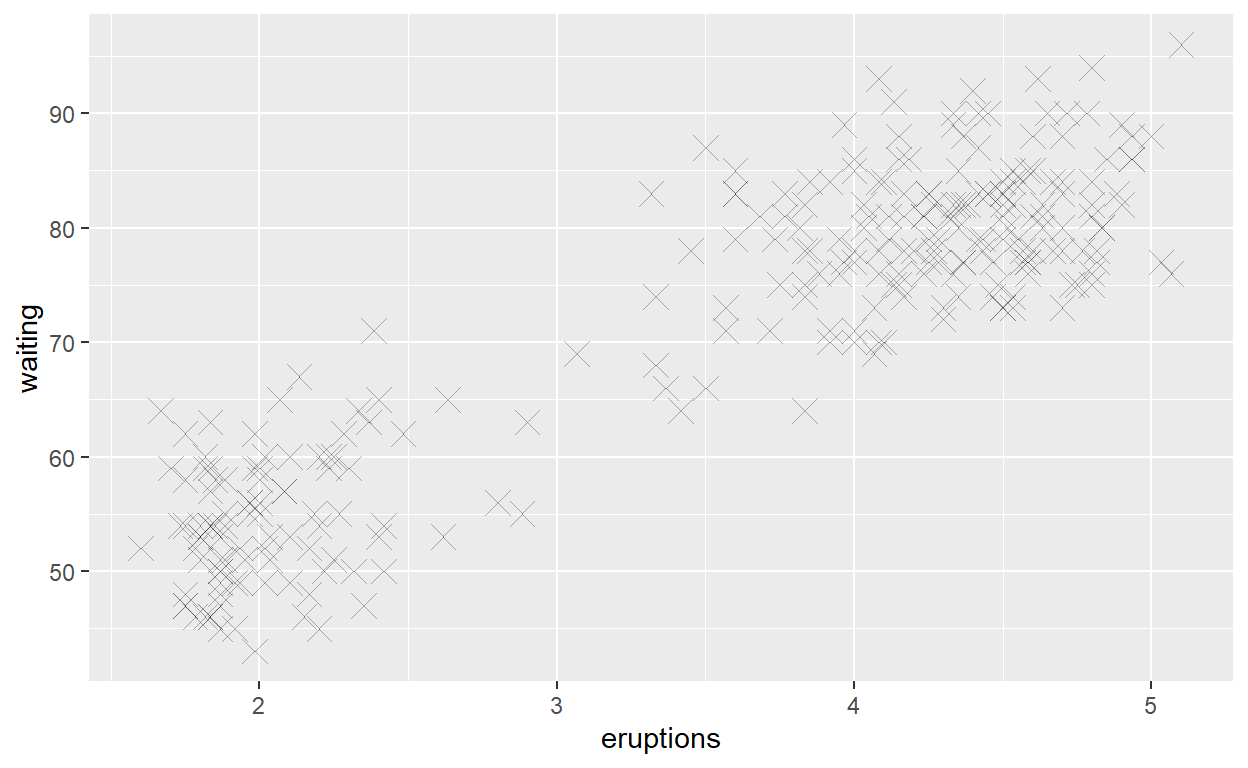# Quiz Q5

``````ggplot(faithful) +
geom_histogram(aes(x = eruptions, fill = eruptions > 3.2))
``````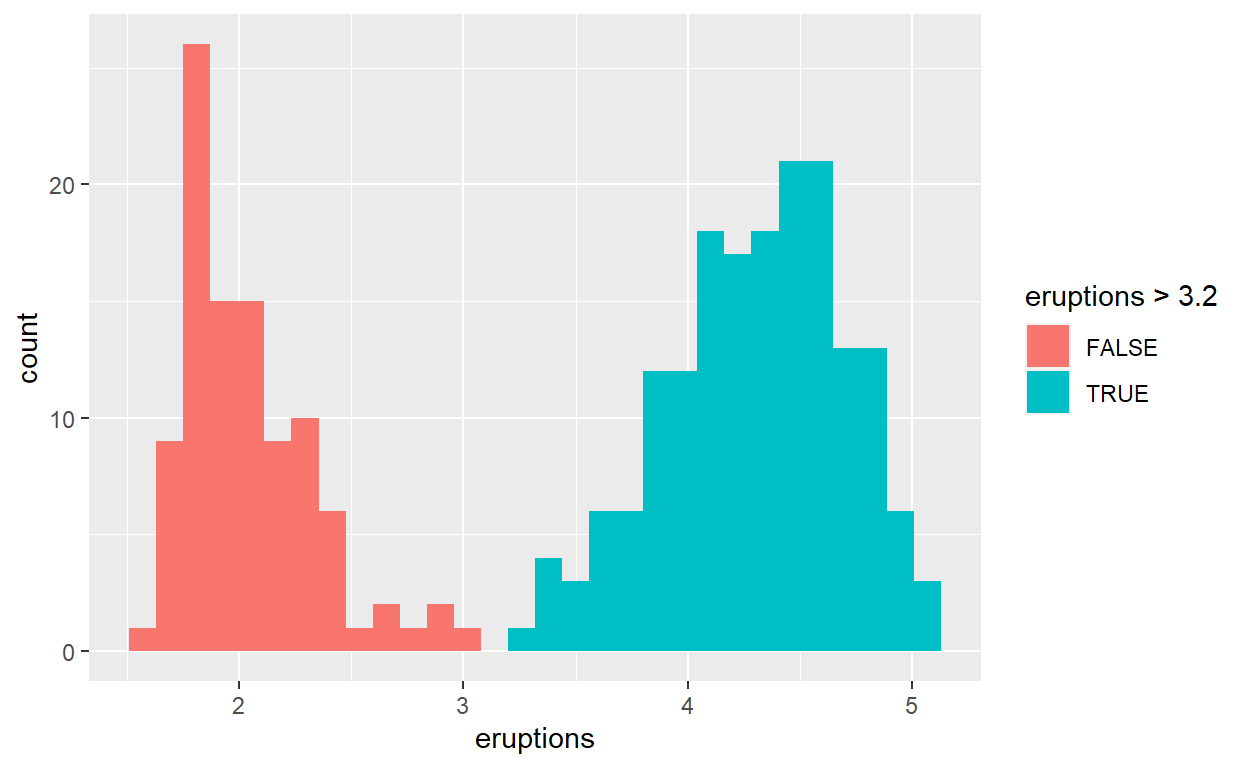# Quiz Q6

``````ggplot(mpg) +
geom_bar(aes(x = manufacturer))
``````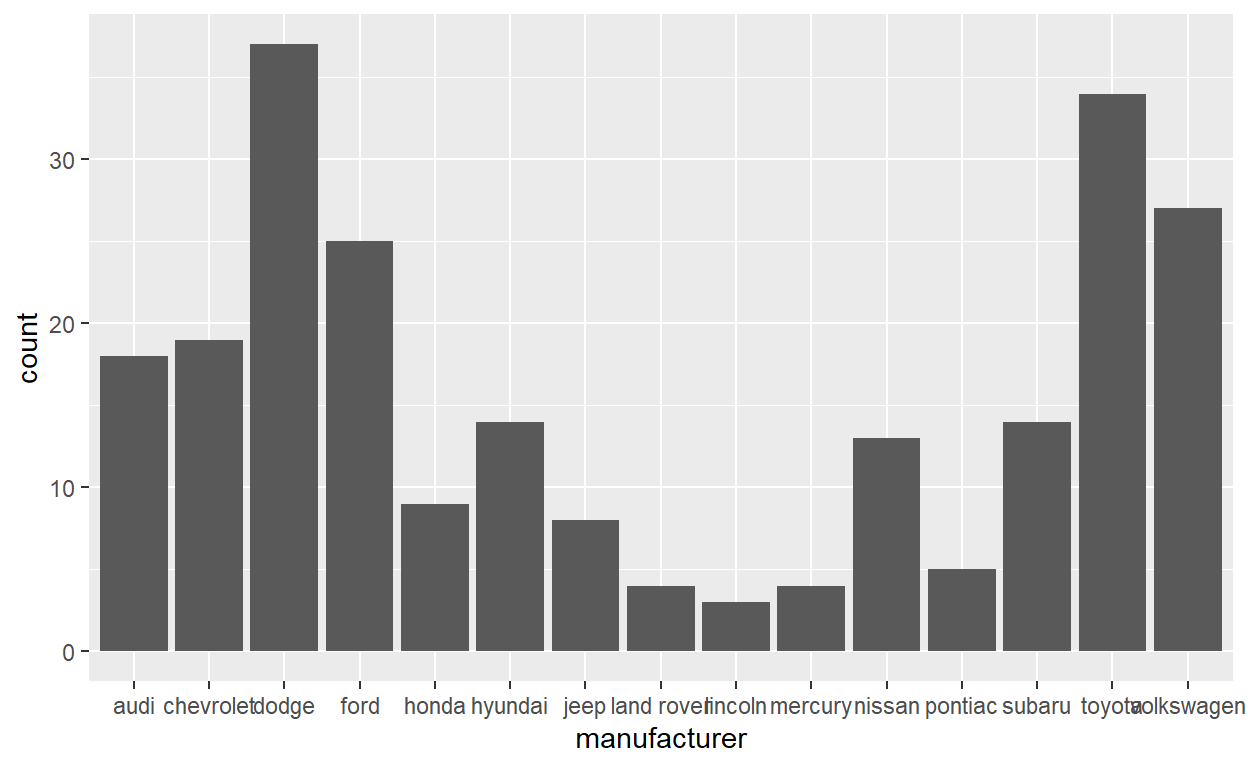# Quiz Q7

``````mpg_counted <- mpg %>%
count(manufacturer, name = 'count')
ggplot(mpg_counted) +
geom_bar(aes(x = manufacturer, y = count), stat = 'identity')
``````# Quiz Q8

``````ggplot(mpg) +
geom_bar(aes(x = manufacturer, y = after_stat(10*count/sum(count))))
``````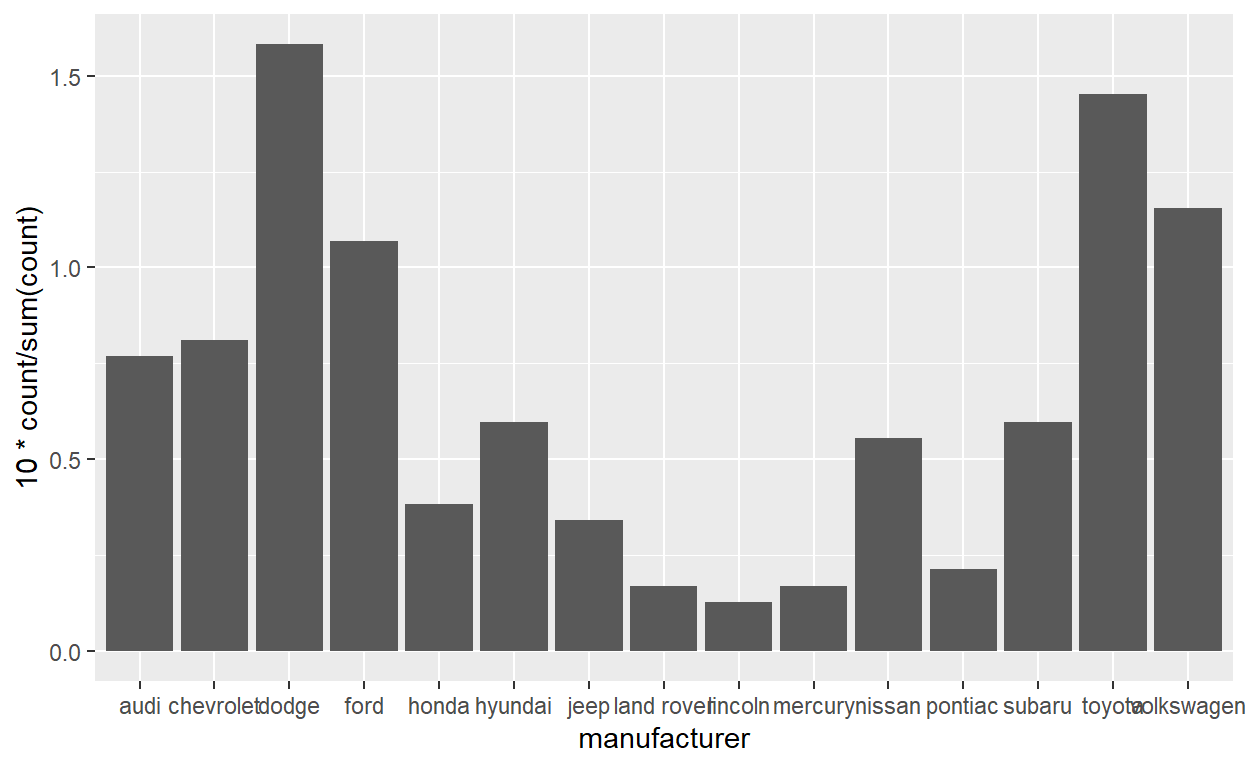Quiz Q9

``````ggplot(mpg) +
geom_jitter(aes(x = class, y = hwy), width = 0.2) +
stat_summary(aes(x = class, y = hwy), geom = "point",
fun = "median", color = "purple",
shape = "asterisk", size = 7)
``````